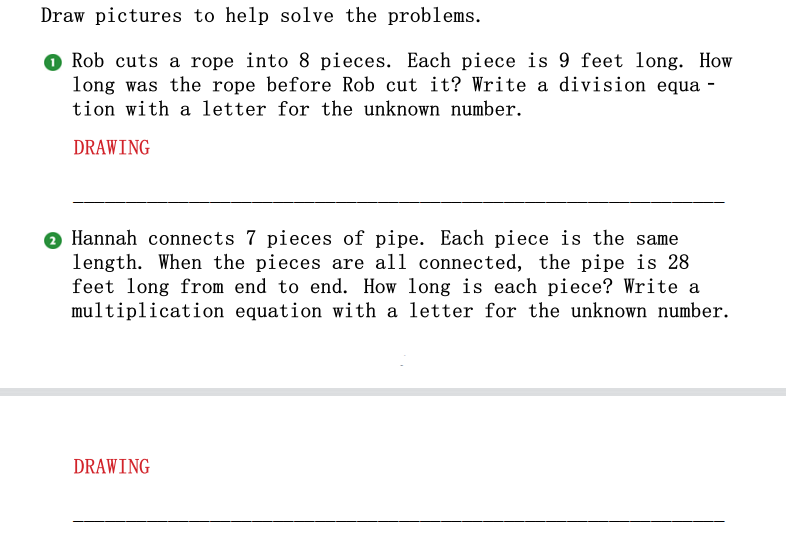### Still have math questions?

Algebra
Question(1) Rob cuts a rope into $$8$$ pieces. Each piece is $$9$$ feet long. How long was the rope before Rob cut it? Write a division equa- tion with a letter for the unknown number.   (2) Hannah connects $$7$$ pieces of pipe. Each piece is the same length. When the pieces are all connected, the pipe is $$28$$ feet long from end to end. How long is each piece? Write a multiplication equation with a letter for the unknown number.